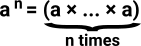# Maths - Category Theory - Exponential Object

If Y and T are objects in a category then, if that category has exponential objects, then YT is also an object in the category. For example cartesian closed categories are an important class of categories that have exponential objects.

We can think of the object YT as representing all functors from T to Y:

T -> Y

Also notated Hom(T,Y) see Hom set on page here.

So exponential objects can have have properties similar to exponents in ordinary algebra.If we think of an exponent as 'n' copies of 'a' then this might be interpreted as a function implemented as a lookup table. an × am =an+m If we interpret a product as a tuple and a sum as 'either' then this equality becomes: (n->a,m->a) = (either (n,m)) -> aIn ordinary number algebra the inverse of the exponential is the logarithm. There doesn't seem to be a direct equivalent of log in category theory but the representables have similar properties.

These equalities from ordinary algebra don't always apply to exponential objects in category theory but they may apply in some reduced form, for instance:

• They may apply 'upto isomorphism'.
• They may apply in simpler categories like sets.

### Example in Set

for instance in the following mapping from set to set:If we are mapping from set T to set Y and the number of elements in T is t and the number of elements in Y is y then the number of mappings from T to Y is yt.

This also behaves like an exponent in that YA * YB = YA+B. That is, if we have 3 categories, one of which is the sum of the other 2, each has a mapping to a 4th category then these mappings will be a product. See diagram on left:For a generalistion of this see Yoneda embedding.

## Evaluation

In propositional logic there is a rule called 'modus ponens':

 B -> A B
A
modus ponens

That is: if 'B implies A' and 'B' is true then A is true.

This has a similar form to this functor:

ε: AB × B -> A

In this case we will call ε 'evaluation'.

Alternativly, for any object C:

f: C × B -> A

there is a unique arrow:

f': C -> AB

The relationship between ε, f and f' is:

ε•(f' × 1B) = f

This is represented by the following diagram:## Relation to Internal Hom-set

An internal hom-set in a cartesian closed category is an exponential object.

## Next Steps

• The exponent category is a Hom set which is discussed on page here.
• Hom sets are used in the page about the Yonada Lemma to relate structures at different levels of abstraction.
• The page here discuses homsets from a set theory perspective.
• Other related pages are:

Catsters youtube videos - Terminal and initial objects

Catsters youtube videos - Products and coproducts

Catsters youtube videos - Pullbacks and pushouts

Catsters youtube videos - General limits and colimits

Where I can, I have put links to Amazon for books that are relevant to the subject, click on the appropriate country flag to get more details of the book or to buy it from them.The Princeton Companion to Mathematics - This is a big book that attempts to give a wide overview of the whole of mathematics, inevitably there are many things missing, but it gives a good insight into the history, concepts, branches, theorems and wider perspective of mathematics. It is well written and, if you are interested in maths, this is the type of book where you can open a page at random and find something interesting to read. To some extent it can be used as a reference book, although it doesn't have tables of formula for trig functions and so on, but where it is most useful is when you want to read about various topics to find out which topics are interesting and relevant to you.